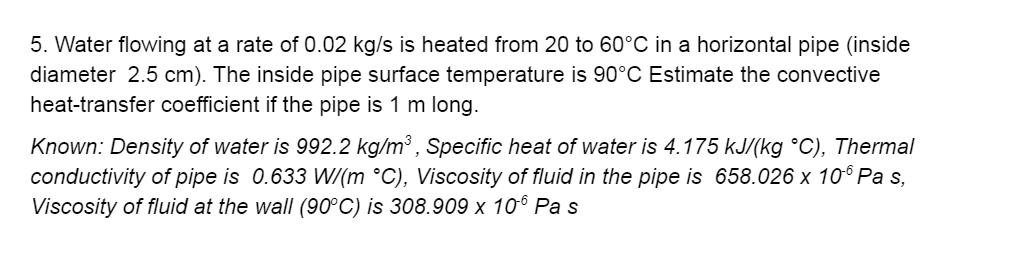# Water flowing at a rate of 0.02 kg/s is heated from 20 to 60°C in a horizontal pipe (inside diameter 2.5 cm). The inside pipe surface temperature is 90°C Estimate the convective heat-transfer coefficient if the pipe is 1 m long. Known: Density of water is 992.2 kg/mº, Specific heat of water is 4.175 kJ/(kg °C), Thermal conductivity of pipe is 0.633 W/(m °C), Viscosity of fluid in the pipe is 658.026 x 106 Pas, Viscosity of fluid at the wall (90°C) is 308.909 x 106 Pas Show transcribed image text 5. Water flowing at a rate of 0.02 kg/s is heated from 20 to 60°C in a horizontal pipe (inside diameter 2.5 cm). The inside pipe surface temperature is 90°C Estimate the convective heat-transfer coefficient if the pipe is 1 m long. Known: Density of water is 992.2 kg/mº, Specific heat of water is 4.175 kJ/(kg °C), Thermal conductivity of pipe is 0.633 W/(m °C), Viscosity of fluid in the pipe is 658.026 x 106 Pas, Viscosity of fluid at the wall (90°C) is 308.909 x 106 Pas

Question-AnswerCategory: Heat And Mass TransferWater flowing at a rate of 0.02 kg/s is heated from 20 to 60°C in a horizontal pipe (inside diameter 2.5 cm). The inside pipe surface temperature is 90°C Estimate the convective heat-transfer coefficient if the pipe is 1 m long. Known: Density of water is 992.2 kg/mº, Specific heat of water is 4.175 kJ/(kg °C), Thermal conductivity of pipe is 0.633 W/(m °C), Viscosity of fluid in the pipe is 658.026 x 106 Pas, Viscosity of fluid at the wall (90°C) is 308.909 x 106 Pas Show transcribed image text 5. Water flowing at a rate of 0.02 kg/s is heated from 20 to 60°C in a horizontal pipe (inside diameter 2.5 cm). The inside pipe surface temperature is 90°C Estimate the convective heat-transfer coefficient if the pipe is 1 m long. Known: Density of water is 992.2 kg/mº, Specific heat of water is 4.175 kJ/(kg °C), Thermal conductivity of pipe is 0.633 W/(m °C), Viscosity of fluid in the pipe is 658.026 x 106 Pas, Viscosity of fluid at the wall (90°C) is 308.909 x 106 Pas

Water flowing at a rate of 0.02 kg/s is heated from 20 to 60°C in a horizontal pipe (inside diameter 2.5 cm). The inside pipe surface temperature is 90°C Estimate the convective heat-transfer coefficient if the pipe is 1 m long. Known: Density of water is 992.2 kg/mº, Specific heat of water is 4.175 kJ/(kg °C), Thermal conductivity of pipe is 0.633 W/(m °C), Viscosity of fluid in the pipe is 658.026 x 106 Pas, Viscosity of fluid at the wall (90°C) is 308.909 x 106 Pas

Show transcribed image text 5. Water flowing at a rate of 0.02 kg/s is heated from 20 to 60°C in a horizontal pipe (inside diameter 2.5 cm). The inside pipe surface temperature is 90°C Estimate the convective heat-transfer coefficient if the pipe is 1 m long. Known: Density of water is 992.2 kg/mº, Specific heat of water is 4.175 kJ/(kg °C), Thermal conductivity of pipe is 0.633 W/(m °C), Viscosity of fluid in the pipe is 658.026 x 106 Pas, Viscosity of fluid at the wall (90°C) is 308.909 x 106 Pas